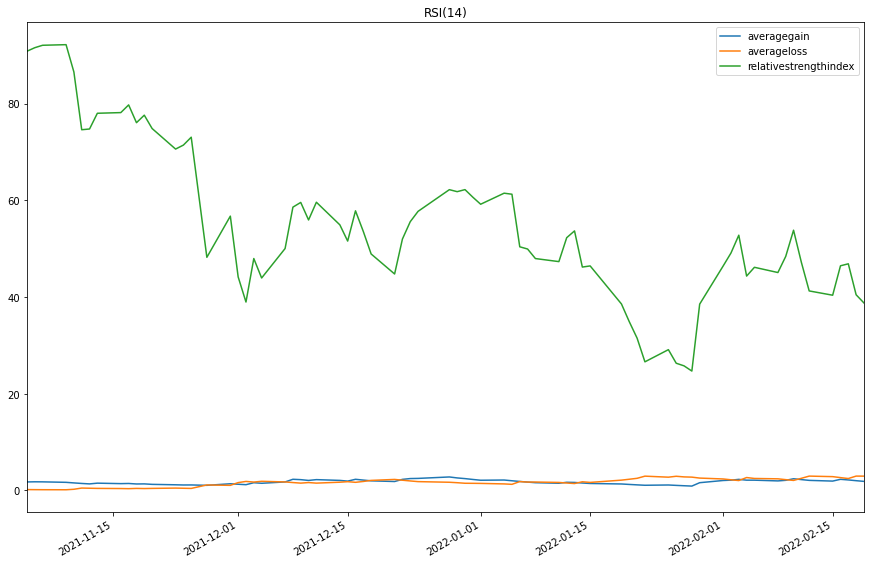# Indicator Reference

## Relative Strength Index

### Introduction

This indicator represents the Relative Strength Index (RSI) developed by K. Welles Wilder. You can optionally specified a different moving average type to be used in the computation

### Create Manual Indicators

You can manually create a RelativeStrengthIndex indicator, so it doesn’t automatically update. Manual indicators let you update their values with any data you choose. The following reference table describes the RelativeStrengthIndex constructor.

### RelativeStrengthIndex()1/2

            RelativeStrengthIndex QuantConnect.Indicators.RelativeStrengthIndex (
int                 period,
*MovingAverageType  movingAverageType
)


Initializes a new instance of the RelativeStrengthIndex class with the specified name and period.

### RelativeStrengthIndex()2/2

            RelativeStrengthIndex QuantConnect.Indicators.RelativeStrengthIndex (
string              name,
int                 period,
*MovingAverageType  movingAverageType
)


Initializes a new instance of the RelativeStrengthIndex class with the specified name and period.

The following table shows the MovingAverageType enumeration members and the indicator that implements each one:

MovingAverageTypeUnderlying Indicator
SimpleSimple Moving Average
ExponentialExponential Moving Average
WildersWilder Moving Average
LinearWeightedMovingAverageLinear Weighted Moving Average
DoubleExponentialDouble Exponential Moving Average
TripleExponentialTriple Exponential Moving Average
TriangularTriangular Moving Average
T3T3 Moving Average
KamaKaufman Adaptive Moving Average
HullHull Moving Average
AlmaArnaud Legoux Moving Average

### Update Manual Indicators

You can update the indicator automatically or manually.

#### Automatic Update

To register a manual indicator for automatic updates with the security data, call the RegisterIndicator method.


private RelativeStrengthIndex _rsi;

// In Initialize()
_rsi = new RelativeStrengthIndex(name, period);
_rsi.Updated += IndicatorUpdateMethod;

RegisterIndicator(symbol, _rsi, Resolution.Daily);

// In IndicatorUpdateMethod()
{
var indicatorValue = _rsi.Current.Value;
}

# In Initialize()
self.rsi = RelativeStrengthIndex(name, period)
self.rsi.Updated += self.IndicatorUpdateMethod

self.RegisterIndicator(symbol, self.rsi, Resolution.Daily)

# In IndicatorUpdateMethod()
indicator_value = self.rsi.Current.Value

To customize the data that automatically updates the indicator, see Custom Indicator Periods and Custom Indicator Values.

#### Manual Update

Updating your indicator manually enables you to control when the indicator is updated and what data you use to update it. To manually update the indicator, call the Update method with time/decimal pair. The indicator will only be ready after you prime it with enough data.


private RelativeStrengthIndex _rsi;
private Symbol symbol;

// In Initialize()
_rsi = new RelativeStrengthIndex(period);

// In OnData()
if (data.ContainsKey(_symbol))
{
_rsi.Update(data[symbol].EndTime, data[symbol].High);
}
{
var indicatorValue = _rsi.Current.Value;
}

# In Initialize()
self.rsi = RelativeStrengthIndex(period)

# In OnData()
if data.ContainsKey(self.symbol):
self.rsi.Update(data[self.symbol].EndTime, data[self.symbol].High)
indicator_value = self.rsi.Current.Value

### Create Automatic Indicators

The RSI method creates an RelativeStrengthIndex indicator, sets up a consolidator to update the indicator, and then returns the indicator so you can use it in your algorithm.

The following reference table describes the RSI method:

### RSI()1/1

            RelativeStrengthIndex QuantConnect.Algorithm.QCAlgorithm.RSI (
Symbol                           symbol,
Int32                            period,
*MovingAverageType               movingAverageType,
*Nullable<Resolution>      resolution,
*Func<IBaseData, Decimal>  selector
)


Creates a new RelativeStrengthIndex indicator. This will produce an oscillator that ranges from 0 to 100 based on the ratio of average gains to average losses over the specified period.

The following table shows the MovingAverageType enumeration members and the indicator that implements each one:

MovingAverageTypeUnderlying Indicator
SimpleSimple Moving Average
ExponentialExponential Moving Average
WildersWilder Moving Average
LinearWeightedMovingAverageLinear Weighted Moving Average
DoubleExponentialDouble Exponential Moving Average
TripleExponentialTriple Exponential Moving Average
TriangularTriangular Moving Average
T3T3 Moving Average
KamaKaufman Adaptive Moving Average
HullHull Moving Average
AlmaArnaud Legoux Moving Average

If you don't provide a resolution, it defauls to the security resolution. If you provide a resolution, it must be greater than or equal to the resolution of the security. For instance, if you subscribe to hourly data for a security, you should update its indicator with data that spans 1 hour or longer.

### Get Indicator Values

To get the value of the indicator, use its Current.Value attribute.

private RelativeStrengthIndex _rsi;

// In Initialize()
_rsi = RSI(symbol, period);

// In OnData()
{
var averageLoss = _rsi.AverageLoss.Current.Value;
var averageGain = _rsi.AverageGain.Current.Value;
var current = _rsi.Current.Value;
}
# In Initialize()
self.rsi = self.RSI(symbol, period)

# In OnData()
average_loss = self.rsi.AverageLoss.Current.Value
average_gain = self.rsi.AverageGain.Current.Value
current = self.rsi.Current.Value


### Visualization

To plot indicator values, in the OnData event handler, call the Plot method.

private RelativeStrengthIndex _rsi;

// In Initialize()
_rsi = RSI(symbol, period);

// In OnData()
{
Plot("My Indicators", "averageloss", _rsi.AverageLoss);
Plot("My Indicators", "averagegain", _rsi.AverageGain);
Plot("My Indicators", "relativestrengthindex", _rsi.Current);
}
# In Initialize()
self.rsi = self.RSI(symbol, period)

# In OnData()